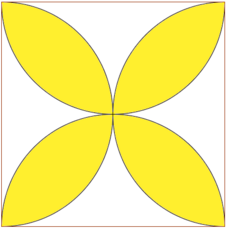Pretty Yellow Petals

Geometry Level 2The figure above shows a square with side length, $a$. Find the area of the yellow region in terms of $a$.

Hint: Circumcentre of each semicircle is midpoint of the square's side.

×